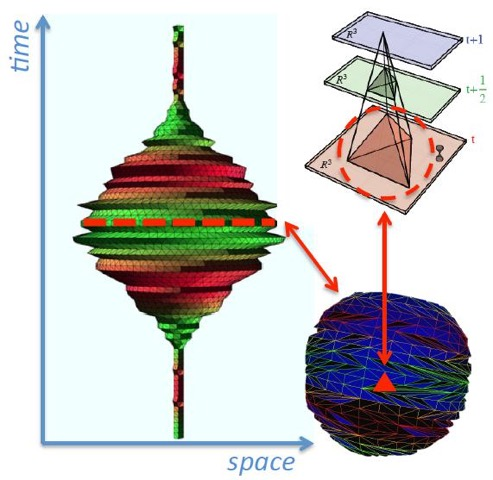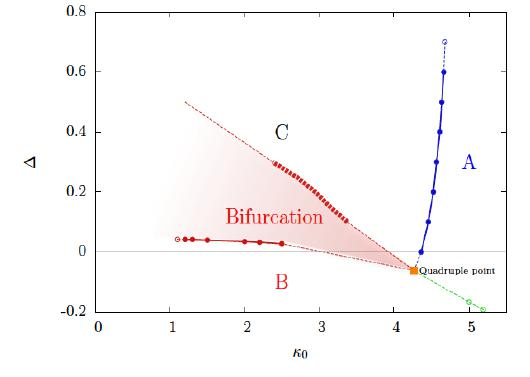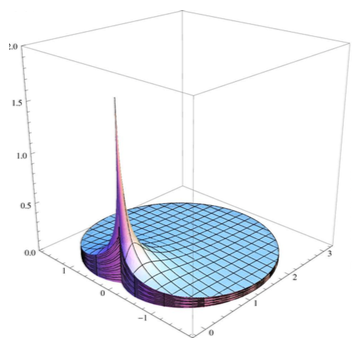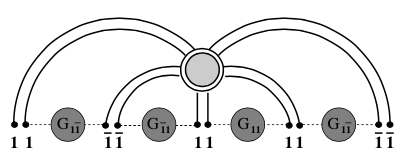## Quantum Gravity and Cosmology

Unifying quantum theory with general relativity to form a complete and predictive theory of quantum gravity is one the great outstanding challenges of modern physics. Here, at the Marian Smoluchowski Institute of Physics our ambitious aim is to achieve this unification via an approach known as Causal Dynamical Triangulations (CDT). A key strength of CDT is the simplicity of construction: we assume only the central principles of Quantum Theory and General Relativity, including few additional ingredients. CDT models continuous spacetime via the connectivity of an ensemble of locally flat d-dimensional triangular building blocks. In close analogy to the sum over all possible paths in Feynman's path integral approach to Quantum Mechanics, CDT sums over all allowed spacetime geometries. A central feature of CDT is the foliation of spacetime into space-like hypersurfaces of fixed topology.

Analytical methods in 4-dimensional Causal Dynamical Triangulations have thus far proved intractable due to the complex nonperturbative sum over geometries. However, with the advent of powerful computational tools, numerical approaches to dynamical triangulations can now be successfully employed via Monte Carlo simulations. Due to this fact the implementation of our research is predominately numerical and utilizes a computational cluster and network of computer nodes within the department.

Despite the simplicity of construction, or perhaps because of it, CDT has produced a number of important results and conceptual insights, and is now widely regarded as a serious contender for a nonperturbative and background independent theory of Quantum Gravity. Some of the successes of the CDT approach include evidence for a classical limit that closely resembles general relativity on large distance scales, while on short distance scales it has yielded some exciting clues as to the nature of space-time at the Planck scale. In particular, there is evidence that 4-dimensional space-time dynamically emerges on large distance scales from a lower dimensional geometry at the Planck scale. This intriguing phenomenon, known as dimensional reduction, has since been observed in a number of other approaches to Quantum Gravity and is an active field of research.Although CDT has enjoyed a number of successes, of course many questions still remain. Does CDT have a well defined continuum limit? Can CDT help us to understand whether dimension and metric signature are fixed or dynamical quantities? Is the introduction of a causality condition via the foliation of space-time an essential ingredient in the formulation of quantum gravity, or can this condition be relaxed? Can CDT help us to understand other approaches to quantum gravity such as Horava-Lifshitz gravity, Loop Quantum Cosmology or asymptotically safe gravity? And ultimately, how can we successfully unite quantum theory with General Relativity? Our research group is actively pursuing answers to these important questions.

## Random Matrix Theory

One can look at random matrix theory (hereafter RMT) as an alternative to classical probability calculus, where instead of single random variable we have to deal with a huge set of random numbers arranged in a matrix-like structure. Such construction is not only possible, but it shares amazing similarities to classical probability calculus. Moreover, this correspondence can be formalized at the mathematical level in the case where the size of the random matrix tends to infinity. By no means this is a severe restriction, since in contemporary applications the sizes of random matrices can easily reach the order of 10^4 (financial engineering), 10^7 (wireless networks) or even 10^10 (genetics). So, almost paradoxically, the bigger the size of the random matrix, the more definite prediction we can make on its statistical properties.

The cornerstone of this new calculus of large matrices is the study of the spectra (eigenvalues) of the matrices. Alike in a classical probability calculus, powerful central limit theorems exist in random matrix theory. This is the reason, why so many similar, macroscopic spectral properties are shared by diverse and unrelated complex random systems (in analogy to the ubiquitous Gaussian distribution in single-valued random structures). We call this phenomenon macroscopic universality of RMT. Macroscopic - as a distinction from the so-called microscopic universality of RMT, a second powerful phenomenon responsible for the wide scope of applications of RMT. Microscopic universality of RMT is the consequence of interactions between the eigenvalues. Since this interaction is long-ranged, certain critical spectral phenomena can emerge locally at the vicinity of some points of the spectra of the matrices. The spectral behavior in the vicinity of these points (fluctuations) depends usually only on the symmetries of the system, therefore can be categorized into universality classes, shared by very different complex systems respecting the underlying symmetry of the matrix model.Random matrix theory (and in particular its version known as Free Random Variables) can be therefore described as the probability calculus of the XXI century. Nowadays, it is hard to find a branch of science where it does not have any applications.

## String Theory and AdS/CFT correspondence

Description in preparation.

## Data Science, Machine Learning and the study of Complex Systems

Description in preparation.

## Other (related) areas of research

• Network Theory
• Quantum Chromodynamics
• Econophysics
• Mathematical methods of theoretical physics applied to interdisciplinary problems
• ...